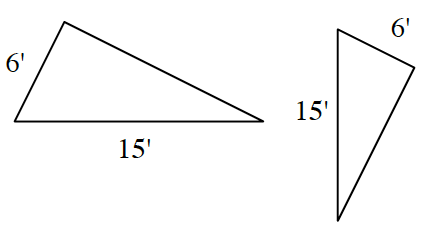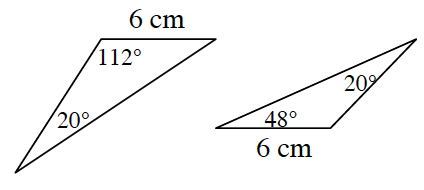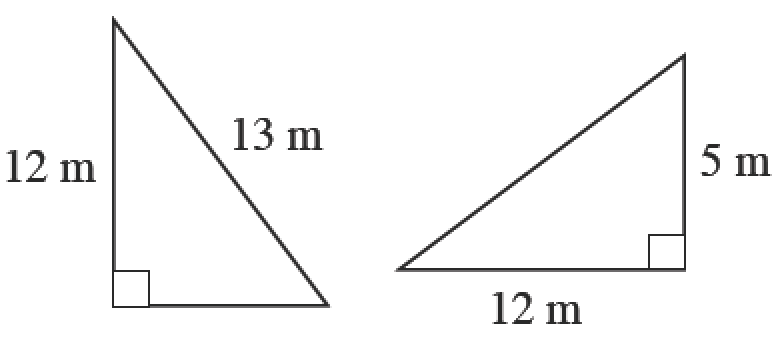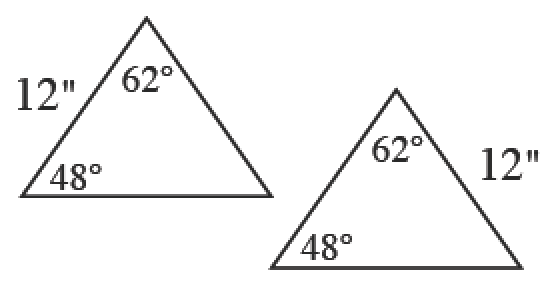### Home > INT1 > Chapter 7 > Lesson 7.2.1 > Problem7-91

7-91.

Which pairs of triangles below are congruent? If the pair is congruent, justify your conclusion by drawing a flowchart and writing an appropriate triangle congruence condition. Note: The diagrams are not necessarily drawn to scale.

In this course you are expected to label your triangles.

What does it mean for two triangles to be congruent?
What does it mean for two triangles to be similar?
How are these two definitions different?

1.Read the Math Notes box in lesson 7.1.7 on triangle congruence conditions. Is there enough information to prove the triangles congruent?

1.Is there a way to determine the missing angles in both triangles?

congruent ($\text{ASA} ≅$ or $\text{AAS} ≅$)

1.Use the Pythagorean Theorem to solve for the missing side.

1.Where is the $12$ located on each triangle?

similar ($\text{AA}{\sim}$) but not congruent since the two sides of length $12$ are not corresponding